NEET  >  Short & Long Answer Questions: Motion in a Plane - 1

# Short & Long Answer Questions: Motion in a Plane - 1 - Physics Class 11 - NEET

Q.1. Why cannot be vectors added algebraically?
Answer. Apart from magnitude, the vectors also have directions, so they cannot be added algebraically.

Q.2. State the essential condition for the addition of vectors.
Answer. The essential condition for the addition of vectors is that they must represent the physical quantities of the same nature.

Q.3. Is time a vector quantity? Give reason.
Answer. No, time flows from past to present and present to the future. Thus the direction of time flow is unique and does not need to be specified. Hence time is not a vector, though it has a direction.

Q.4. Is pressure a vector? Give reason.
AnswerNo, The pressure is always taken to be normal to the plane of the area on which it is acting. As this direction is unique, it does need any specification. So pressure is not a vector.

Q.5. Can two vectors of different magnitudes be combined to give zero resultant?
Answer. No, two vectors of different magnitudes cannot be combined to give zero resultant. This is because the effect of vectors cancels out only when they act in the opposite direction and have the same magnitude.

Q.6. When is the magnitude of the resultant of two vectors equal to either of them?
Answer. When two vectors of equal magnitude are inclined to each other at an angle of 1200, the magnitude of their resultant is equal to that of either vector.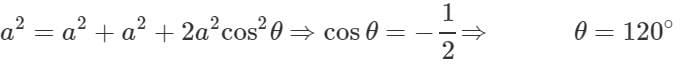Q.7. Are the magnitude and direction of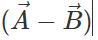same as that of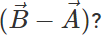Answer. The vectors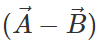and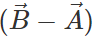have equal magnitude but opposite directions.

Q.8. Can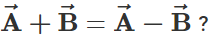Answer. Yes. The equality holds when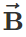is a null vector.

Q.9. When is the magnitude of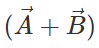equal to the magnitude of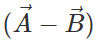?
Answer. When the vectors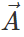and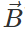are perpendicular to each other,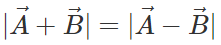Q.10 Under what condition will the directions of sum and difference of two vectors be same?

Answer: When the two vectors are unequal in magnitude and are in the same direction.

Q.11 Can we add a velocity vector to a displacement vector?

Answer: No, only vectors representing physical quantities of same nature can be added together.

Q.12  What is the minimum number of coplanar vectors of different magnitudes which can give zero resultant?

Answer: Three. If three vectors can be represented by the three sides of a triangle taken in the same order, then their resultant is a zero vector.

Q.13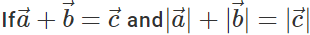what can we say about the direction of these vectors?

Answer: The three vectors have the same direction

Q.14 What is the resultant of vector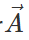multiplied by real number m?

Answer: The resultant vector m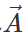has magnitude m times that of. It has same direction as that ofif m is positive. It has a direction opposite to that ofif m is negative.

Q.15 Can a vector be multiplied by both dimensional and non-dimensional scalars?

Answer: Yes. When a vector is multiplied by a dimensional scalar, the resultant has different dimensions. When the vector is multiplied by a non-dimensional scalar, its dimensions remain unchanged.

Q.16 What is the maximum number of components into which a vector can be resolved?

Q.17 If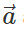and Δare directed opposite to each other, what is the relation between Δand |Δ|?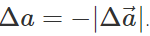Q.18 Give two conditions necessary for a given quantity to be a vector.

Answer: (i) The quantity must have both magnitude and direction.
(ii) It must obey the laws of vector addition.

Q.19 Is finite rotation a vector?

Answer: No. This is because the addition of two finite rotations about different axes does not obey commutative law.

Q.20 Can the scalar product of two vectors be negative?

Answer: Yes, the scalar product is negative when the angle between two vectors lies between 900  and 2700.

Q.21 What is the dot product of two per-perpendicular vectors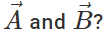Answer: Zero, as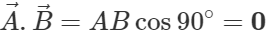Q.22 What is the dot product of two similar unit vectors?

Answer: Unity. For example,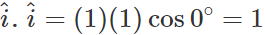Q.23 What is the dot product of two dissimilar unit vectors? Or Calculate the value of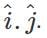Answer: Zero. For example,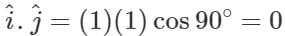Q.24 If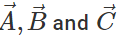are non-zero vectors and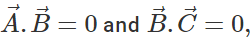then find out the value of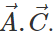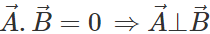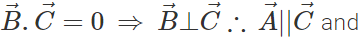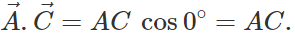Q.25 What is the magnitude and direction of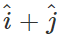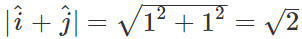If the vector i^+j^ makes angle β with x-axis, then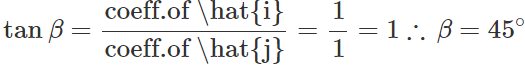Q.26 What is the angle made by vector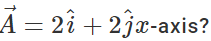The angle θ between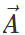and x-axis is given by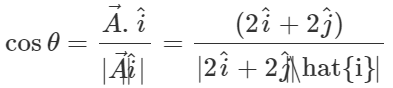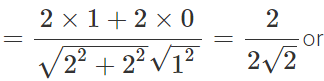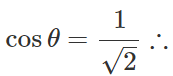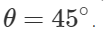Q.27 What should be the angle θ between two vectors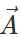and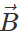for their resultant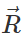to be maximum?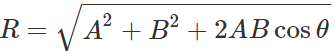R will be maximum when cosθ=+1 or θ=0∘   Rmax=A+B

Q.28 What is the effect on the magnitude of the resultant of two vectors when the angle θ between them is increased from 00 to 1800?
As the angle θ increases from 00  to 1800, the value of cosθ decreases, so the magnitude R of the resultant also decreases.

Q.29 Two persons are pulling the ends of string in such a way that the string is stretched horizontally. When a weight of 10 kg is suspended in the middle of the string, the string does not remain horizontal. Can the persons make it horizontal again by putting it with a greater force?

Answer: No, the vertical weight cannot be balanced by the horizontal force, however large the two forces may be.

Q.30 What is the vector sum of n co-planar forces, each of magnitude F, if each force makes an angle of 2π/n with the preceding force?

Answer: Total angle between n coplanar forces =(2π/n)×n=2π. This shows that forces can be represented by the n sides of a closed polygon taken in the same order. So the resultant force is zero.

Q.31 If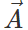and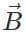are two length vectors, then what is the geometrical significance of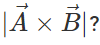Answer: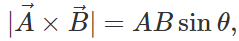this gives area of the parallelogram with adjacent sidesand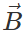.

Q.32 What is the unit vector perpendicular to the plane of vectors?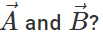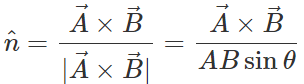Q.33 What is the value of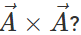Answer: Zero, because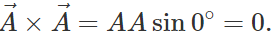Q.34 What is the condition for two vectors to be collinear?

Answer: For two given vectors to be collinear, their cross-product must be zero.

Q.35 Find the value of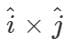.

Answer:=(1)(1) sin90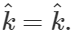Q.36 What is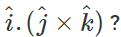Answer: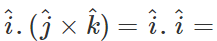(1)(1)cos0=1

Q.37 Under what condition will the equality: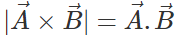hold good?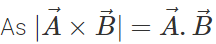∴  ABsinθ=ABcosθ or tanθ=1
⇒θ=45.

Q.38  What is the angle between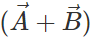and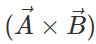?

Answer: The resultant vectorlies in the plane of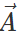and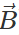, while the cross productis perpendicular to this plane. So the angle betweenandis 90.

Q.39 Can there be motion in two dimensions with acceleration only in one dimension?

Answer: Yes, in a projectile motion, the acceleration acts vertically downwards while the projectile follows a parabolic path.

Q.40 Can the direction of velocity of a body change when the acceleration is constant?

Answer: Yes, in projectile motion the direction of velocity changes from point to point, but its acceleration is always constant and acts vertically downwards.

Q.41 Is the rocket in flight an example of projectile?

Answer: No, because it is propelled by combustion of fuel and does not move under the effect of gravity alone.

Q.42 A stone is thrown vertically upwards and then it returns to the thrower. Is it a projectile?

Answer: It is not a projectile, because a projectile should have two-component velocities in two mutually perpendicular directions, but in this case, the body has velocity only in one direction while going up or coming downwards.

Q.43 Why does a projectile fired along the horizontal not follow a straight line path?

Answer: Because the projectile fired horizontally is constantly acted upon by acceleration due to gravity acting vertically downwards.

Q.44 A body projected horizontally moves with the same horizontal velocity although it is under the action of force of gravity. Why?

Answer: The force of gravity acts in the vertically downward direction and has no effect on the horizontal component of velocity, and this makes the body to move with constant horizontal velocity.

Q.45 What is the angle between the direction of velocity and acceleration at the highest point of a projectile path?

Answer: 90. At the highest point, the vertical component of velocity becomes zero, the projectile has only a horizontal velocity while the acceleration due to gravity acts vertically downwards.

Q.46 A bullet is dropped from a certain height and at the same time, another bullet is fired horizontally from the same height. Which one will hit the ground earlier and why?

Answer: Since the heights of both bullets from the ground are the same, the time taken by both of them to reach the ground will be the same.

Q.47 A stone dropped from the window of a stationary bus takes 5 seconds to reach the ground. In what time the stone will reach the ground when the bus is moving with (a) constant velocity of 80 kmh−1 (b) constant acceleration of 2 kmh−2 ?

Answer: (a) 5 seconds (b) 5 seconds.
In both cases, initial vertical velocity is zero, downward acceleration is equal to ′g′ and also vertical distance covered is same.

Q.48 A bomb thrown as projectile explodes in mid-air. What is the path traced by the center of mass of the fragments assuming the friction to be negligible?

Answer: The path traced by the center of mass of the fragments is a parabola.

Q.49 At what point in its trajectory does a projectile have its (i) minimum speed (ii) maximum speed?
For a projectile given angular projection, the horizontal component of velocity remains constant throughout while the vertical component first decreases, becomes zero at the highest point and then increases again. Hence
(i) Projectile has minimum speed at the highest point of its trajectory.
(ii) Projectile has maximum speed at the point of projection and at the point where it returns to the horizontal plane of projection.

Q.50 What will be the effect on horizontal range of a projectile when its initial velocity is doubled, keeping the angle of projection same?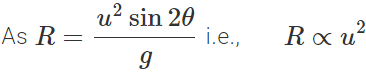When u is doubled, the horizontal range becomes four times the original horizontal range.

Q.51 A projectile is fired at an angle of 15 to the horizontal with the speed υ. If another projectile is projected with the same speed, then at what angle with the horizontal it must be projected so as to have the same range?

Answer: For the same R and υ, the sum of the two angles of projection is 90. As one angle is of 15, others should be 90−15 =75.

Q.52 Is the maximum height attained by projectile is largest when its horizontal range is maximum?

Answer: No. Horizontal range is maximum when θ=45 and maximum height attained by projectile is largest when θ=90.

Q.53 What will be the effect on maximum height of a projectile when its angle of projection is changed from 30 to 60, keeping the same initial velocity of projection?

Answer: Maximum height,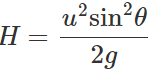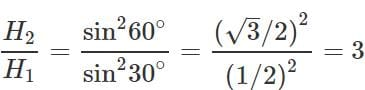Thus the maximum height becomes three times the original maximum height.

Q.54 A body is projected with speed u at an angle θ to the horizontal to have maximum range. What is the velocity at the highest point?

Answer: For maximum horizontal range, θ=45 Velocity at highest point = Horizontal component of velocity =u cos 45=u/√2

Q.55 What is the angle of projection for a projectile motion whose range R is n times the maximum height H?

Answer: Here    R=nH or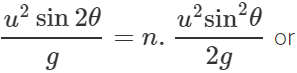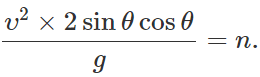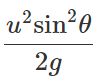ortanθ = 4/n   θ=tan−1(4/n)

Q.56  The greatest height to which a man can throw a stone is h. What will be the greatest distance up to which he can throw the stone?
Answer: Maximum height attained,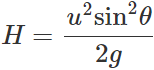Clearly, H will be maximum when sinθ=1
∴  Hmax= u2/g =2h.

Q.57 A particle is projected at an angle θ from the horizontal with kinetic energy K. What is the kinetic energy of the particle at the highest point?

Answer: If the particle is projected with velocity υ, then its initial kinetic energy is K=1/2 mυ2 Velocity of the projectile at the highest point =υcosθ
∴K.E. of the particle at the highest point = 1/2 m(υcosθ)2=(1/2 mυ2)cos2θ=Kcos2θ.

Q.58 A man can jump on the moon six times as high as on the earth. Why?

Answer: Maximum height attained on earth,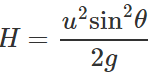Acceleration due to gravity on moon, g′=g/6
∴ Maximum height attained on moon: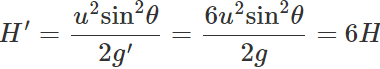So, one can jump on moon six times as high as on earth.

Q.59 A projectile of mass m is fired with velocity υ at an angle θ with the horizontal. What is the change in momentum as it rises to the highest point of the trajectory?

Answer: Horizontal component of velocity remains constant. The vertical component is υ sin θ at the point of projection and zero at the highest point. Magnitude of change in momentum =m(υsinθ−0)=mυsinθ (in the vertical direction).

Q.60 A projectile of mass m is thrown with velocity υ from the ground at an angle of 45 with the horizontal. What is the magnitude of change in momentum between leaving and arriving back at the ground?

Answer: The vertical component of velocity at the point of projection is υ sin θ and at the point of return is −υ sin θ.
∴ Magnitude of change in momentum =m(υsinθ+υsinθ)= 2mυ sinθ = 2mυ sin45=√2mυ (in the vertical direction).

The document Short & Long Answer Questions: Motion in a Plane - 1 | Physics Class 11 - NEET is a part of the NEET Course Physics Class 11.
All you need of NEET at this link: NEET

## FAQs on Short & Long Answer Questions: Motion in a Plane - 1 - Physics Class 11 - NEET

 1. What is motion in a plane?Answer: Motion in a plane refers to the movement of an object in two dimensions, usually represented by the x-axis and y-axis. It involves both displacement and direction.
 2. How is motion in a plane different from motion in a straight line?Answer: Motion in a plane involves movement in two dimensions, while motion in a straight line only occurs in one dimension. In motion in a plane, objects can move both horizontally and vertically.
 3. What are the different types of motion in a plane?Answer: The different types of motion in a plane include rectilinear motion, curvilinear motion, circular motion, projectile motion, and rotational motion. Each type of motion has its own characteristics and equations.
 4. How can we calculate displacement in motion in a plane?Answer: Displacement in motion in a plane can be calculated using the Pythagorean theorem. If an object moves horizontally a distance of "x" and vertically a distance of "y," the displacement can be calculated as √(x^2 + y^2).
 5. What is projectile motion?Answer: Projectile motion is a type of motion in a plane where an object is launched into the air and moves along a curved path under the influence of gravity. It follows a parabolic trajectory and is influenced by both horizontal and vertical components of velocity.

## Physics Class 11

130 videos|472 docs|210 tests

## Physics Class 11

130 videos|472 docs|210 tests

### How to Prepare for NEET

Read our guide to prepare for NEET which is created by Toppers & the best Teachers
Signup to see your scores go up within 7 days! Learn & Practice with 1000+ FREE Notes, Videos & Tests.
10M+ students study on EduRev
Track your progress, build streaks, highlight & save important lessons and more!(Scan QR code)
Related Searches

,

,

,

,

,

,

,

,

,

,

,

,

,

,

,

,

,

,

,

,

,

;